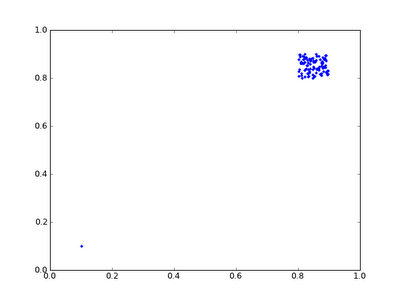## Thursday, 16 June 2011

### That's not normal for a correlation - Pearson vs Spearman

Let me present some random data, 100 data points with x and y values chosen from a uniform distribution between 0.8 and 0.9:Correlation coefficients: -0.074 (Pearson), -0.073 (Spearman)

Now let's add a single data point at 0.1, 0.1:Correlation coefficients: 0.866 (Pearson), -0.042 (Spearman)

Here's another interesting situation, with two random datasets of 50 datapoints chosen from the intervals (0.0, 0.5) and (0.5, 1.0):Correlation coefficients: 0.668 (Pearson), 0.711 (Spearman)

What happens when we contract the areas though?Correlation coefficients: 0.995 (Pearson), 0.719 (Spearman)